Next: Mutual predictability minimisation Up: Previous algorithms for sparse Previous: Previous algorithms for sparse

# Reconstruction error minimisation

We shall first consider the algorithms based on reconstruction error minimisation. Let us denote the inputs to the network by, and the outputs of the network by. Then the network can be described by a mapping from the inputs to the outputs: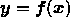. The reconstruction of the input is then a mapping from the outputs to the inputs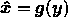. We shall assume that some information is lost and the reconstruction is not exact (cf. pseudoinverse of a matrix). A reasonable way to measure the goodness of the representation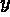is to measure the reconstruction error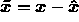with a given error function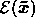. Usually a quadratic reconstruction erroris used due to its mathematical tractability. Figure 2.1 illustrates the architecture underlying unsupervised learning models based on reconstruction error minimisation.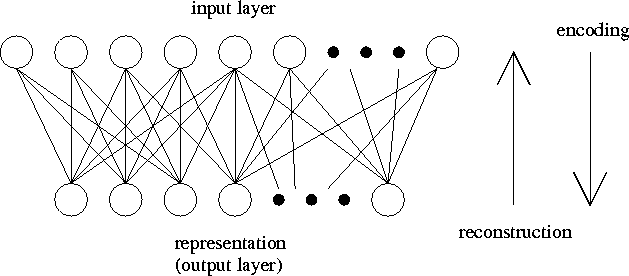Figure 2.1: Architecture underlying unsupervised learning models based on reconstruction error minimisation.

Due to the information loss in mapping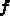there is no unique way to choose the reconstruction mapping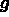. This can be illustrated by considering a linear mapping from 2-dimensional inputto 1-dimensional output y. The mappingis many-to-one, and therefore it does not have a unique inverse. If we are given the output y, there are many candidate inputswhich could have generated the output, thus we have many possible choices for the reconstruction mapping. There is no obvious way to know which one of them would minimise the reconstruction error. If the reconstruction mappingis given instead of, however, we can simply define the mappingto be the one that minimises the reconstruction error. Among all different possible outputs we choose the one that minimises the reconstruction error.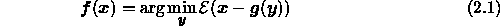In our example this would mean that we first define a linear reconstruction mapping from y toand then solve thethat minimises the quadratic reconstruction error. It turns out that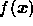is a unique linear mapping.

The example shows how it is often difficult to find the reconstruction mappingfor a given mapping, but equation 2.1 makes it easy to find the mappingfrom a given reconstruction mapping. By using equation 2.1 it is also easy to impose various constraints on the outputs, because we can restrict the search for the minimum in equation 2.1 to the set of allowed outputs.

A further advantage of using equation 2.1 is that the derivatives of the reconstruction error with respect to the outputs are zero because the outputs minimise the reconstruction error. This greatly simplifies the derivation of the learning rule for the network. If we denote the parameters of the network by, then, for a given input, the reconstruction error is a function of the outputs and the weights: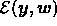. The goal of the learning is to adapt the parameters so that the average reconstruction error made by the network is minimised. We can derive the adaptation rule for the weights by applying gradient descent. To do this we have to be able to compute the derivatives ofwith respect to the weights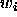.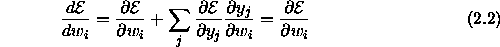The last step follows from the fact that in equation 2.1 the outputs are defined to minimise the reconstruction errorand thus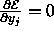for all j. The last step holds even if there were some constraints for the outputs, which do not depend on the parameters. Let us denote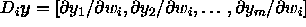. This vector points to the direction of the change in the output, when the parameterchanges by an infinitesimal amount. If the outputs are constrained,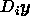can only point to a direction whereis allowed to change. The sum in equation 2.2 can be interpreted as the directional derivative ofin the direction. The outputs minimiseand therefore the derivative is zero in all allowed directions of change of the outputs.

The outputs could, for example, be restricted to be non-negative. Suppose that the outputwould be zero due to this constraint. If the output had been allowed to be negative, the reconstruction error could have been further decreased by decreasing. This means that the derivative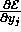cannot be zero. This time, however, the outputwould not change if the parameters changed only by an infinitesimal amount. The outputwould still remain exactly zero. Therefore, in this example it always holds that eitheror.

Problems may arise if equation 2.1 has degenerate solutions. If we are using the network in feedforward phase, that is, we are not adapting the parameters, then we can just pick one of the solutions. We might run into problems if we try to adapt the network, because the mappinghas discontinuities where the equation 2.1 has degenerate solutions, and it is not possible to compute the derivatives of outputsin equation 2.2. Luckily in most practical cases the probability of degenerate solutions is zero.

Equations 2.1 and 2.2 provide us a general framework for developing neural networks based on reconstruction error minimisation. The development starts by defining the reconstruction error, the parametrised reconstruction mapping, and possible constraints for the outputs. Sometimes we are able to solve equation 2.1 in a closed form, but in general we have to solve it numerically.

We shall first consider a simple case where we can find the solution to equation 2.1 in a closed form. If we require the reconstructionto be a linear mapping and there are no constraints imposed on the outputs, it turns out that, assuming the quadratic reconstruction error, also the mappingwill be linear. There are many degrees of freedom in the mapping and we can require the mapping to be orthogonalwithout any loss of generality. The principal component analysis (PCA) gives this kind of orthogonal mapping which is optimal with respect to the quadratic reconstruction error (see e.g. Oja, 1995). The code is maximally dense in the sense that all the neurons participate in representing any given input. (For more information about PCA see the Master's Thesis of Jaakko Hollmén).

If we require the reconstruction to be a linear mapping and furthermore we require the outputsto be binary and exactly one neuron to be active at any one time, then we have made sure that the code we obtain is maximally local. The resulting coding scheme is called vector quantisation (VQ) (see e.g. Abut, 1990), because we can simply associate each neuron with the corresponding reconstruction and then decide the active neuron by finding the neuron whose reconstruction vector is closest to the input according to equation 2.1. This can be interpreted as quantifying the input.

When the same basic scheme can produce both maximally dense and local codes, it is obvious to try to produce also sparse codes. Saund (1994, 1995) has proposed a few different reconstruction mappings, which can be used for sparse coding. They are chosen so that the resulting coding is sparse whenever the input is sparse, i.e., is describable with a sparse code. Unfortunately the inputs have to be binary, which restricts the use of the algorithm. It might be possible to generalise the approach for graded inputs, but it does not seem very easy. Olshausen and Field (1995) have developed an algorithm which uses the same kind of linear reconstruction scheme as PCA and VQ, but have added an extra term which promotes sparsity to the reconstruction error function. Equation 2.1 does not have a simple closed form solution in either of these algorithms, and the outputs have to be computed iteratively by gradient descent of the reconstruction error. This makes the computational complexity depend quadratically on the number of the neurons in the network.

Although the mappingsandcan be related by using equation 2.1, it is also possible to parametrise and learn them both separately. Algorithms using this kind of autoencoder framework have been proposed by several authors, and there has also been modifications which yield sparse codes [Dayan and Zemel, 1995, Zemel, 1993, Hinton and Zemel, 1994]. These algorithms can compute the outputs without any time consuming iterations, but unfortunately learning seems to be very slow. This is probably due to the fact that the network has to learn the same things twice as the learning does not take into account the relation betweenandin any way.Next: Mutual predictability minimisation Up: Previous algorithms for sparse Previous: Previous algorithms for sparse

Harri Lappalainen
Thu May 9 14:06:29 DST 1996